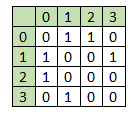# Construct a graph from given degrees of all vertices in C++

Suppose we have a list of vertices, and their degrees are given. We have to generate one undirected graph from that degree sequence. It will not include loop or multiple edges. So if the degree sequence is like [2, 2, 1, 1], then the graph can be likeTo solve this, we will follow these steps −

• for each vertex i, do

• for each vertex j that is valid, and next to i

• if the degree of vertex i and j are more than zero, then connect them

• display the matrix.

## Example

Live Demo

#include <iostream>
#include <iomanip>
using namespace std;
void generateGraph(int vert_degree[], int n) {
for(int i = 0; i<n; i++){
for(int j = 0; j < n; j++){
}
}
for (int i = 0; i < n; i++) {
for (int j = i + 1; j < n; j++) {
if (vert_degree[i] > 0 && vert_degree[j] > 0) {
vert_degree[i]--; vert_degree[j]--;
}
}
}
cout << endl << setw(3) << " ";
for (int i = 0; i < n; i++)
cout << setw(3) << "(" << i << ")";
cout << endl << endl;
for (int i = 0; i < n; i++) {
cout << setw(4) << "(" << i << ")";
for (int j = 0; j < n; j++)
cout << endl;
}
}
int main() {
int vert_degree[] = { 2, 2, 1, 1, 1 };
int n = sizeof(vert_degree) / sizeof(vert_degree);
generateGraph(vert_degree, n);
}



### Output

      (0)   (1)  (2)  (3)  (4)

(0)    0    1    1    0    0
(1)    1    0    0    1    0
(2)    1    0    0    0    0
(3)    0    1    0    0    0
(4)    0    0    0    0    0

Updated on: 03-Jan-2020

181 Views